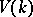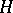# Borel fixed-point theorem

(diff) ← Older revision | Latest revision (diff) | Newer revision → (diff)
A connected solvable algebraic groupacting regularly (cf. Algebraic group of transformations) on a non-empty complete algebraic varietyover an algebraically-closed fieldhas a fixed point in. It follows from this theorem that Borel subgroups (cf. Borel subgroup) of algebraic groups are conjugate (The Borel–Morozov theorem). The theorem was demonstrated by A. Borel . Borel's theorem can be generalized to an arbitrary (not necessarily algebraically-closed) field: Letbe a complete variety defined over a fieldon which a connected solvable-split groupacts regularly, then the set of rational-pointsis either empty or it contains a point which is fixed with respect to. Hence the generalization of the theorem of conjugation of Borel subgroup is: If the fieldis perfect, the maximal connected solvable-split subgroups of a connected-defined algebraic groupare mutually conjugate by elements of the group of-points of.